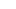# CAT Quant: Solving Tougher Questions from Sequences and Series

(Photo: Erik Abderhalden)

By the end of this month, voucher sales for the CAT would start. That is traditionally what pushes people get really serious about their CAT preparation and coaching institutes start selling fast-track programs, crash courses, etc. I am sure you would encounter posts and tips about “How to prepare for the CAT in 100 days” soon. As a matter of fact, I am considering writing one myself. I have always believed that to reach your peak performance in CAT – you need to prepare for approximately 300 to 500 hours. So, if you haven’t started by now – you might be pushing your luck.

Be that as it may, for this post I am making as assumption that you would know the basics of the Sequence and Series or Progressions topic in quantitative aptitude. We are going to discuss the following ideas in this post,

a) Arithmetico-Geometric Progression

b) Method of Differences

Arithmetico-Geometric Progression (AGP)

An AGP is when terms of an Arithmetic Progression (AP) are multiplied with the corresponding terms of a Geometric Progression (GP).

Consider an AP => a, a + d, a + 2d, a + 3d …

Consider a GP => b, br, br2, br3

The AGP would be => ab, (a + d)br, (a + 2d)br2

Questions based on AGP would typically ask you to find out a particular term or the sum till a particular term in the progression. Let us look at couple of them.

Q1. Find the sum of the series 1*2, 2*22, 3*23…. 50*250

We are trying to find out the sum. Let it be S.

S = 1*2 + 2*22 + 3*23 … 50*250

=> 2S = 1*22 + 2*23 + 3*24 … + 49*250 + 50*251 (shift the terms by 1)

=> S – 2S = 1*2 + 1*22 + 1*23 …. 1*250 – 50*251

=> -S = 2(250 – 1)/(2 – 1) – 50*251

=> -S = 251 – 2 – 50*251

=> -S = -49*251 – 2

=> S = 49*251 + 2

Q2. Find the sum of the series 1 + 7/5 + 13/25 + 19/125 … to infinite terms.S = 25/8

Method of Differences

If the difference of the terms is same at level ‘1’, then the nth term would be equation in ‘n’ of degree 1. If the difference of the terms is same at level ‘2’, then the nth term would be equation in ‘n’ of degree 2.

Let us have a look at it with the help of couple of examples.

Q1. Find out sum of first 20 terms of the series = 1, 3, 5, 7, 9 …

I know that this is a simple AP and you can solve it easily but I am using this series to explain the method.

We are given the series 1, 3, 5, 7, 9…

Difference of terms at Level 1 = 2, 2, 2, 2, 2. This is a constant

=> nth term would be an equation in n of degree 1.

=> T(n) = an + b

Now comes the question of finding out the values of ‘a’ and ‘b’. For that we need two equations in ‘a’ and ‘b’. We can get those by putting n = 1 and 2Q2. Find out sum of first 20 terms of the series = 6, 11, 18, 27, 38, 51…

Difference of terms at Level 1 = 5, 7, 9, 11, 13

Difference of terms at Level 2 = 2, 2, 2, 2. This is a constant.

=> nth term would be an equation in n of degree 2

=> T(n) = an2 + bn + c

Now comes the question of finding out the values of ‘a’ and ‘b’. For that we need two equations in ‘a’, ‘b’ and ‘c’. We can get those by putting n = 1, 2 and 3.Another tip that I would like to give you is that if T(n) is an equation of degree 2, the coefficient of n2 will be half of the constant difference.

I hope you found this post useful.

Ravi Handa has taught Quantitative Aptitude at various coaching institutes for seven years. An alumnus of IIT Kharagpur where he studied a dual-degree in computer science, he currently runs an online CAT 2013 coaching course and the website Handa Ka Funda.